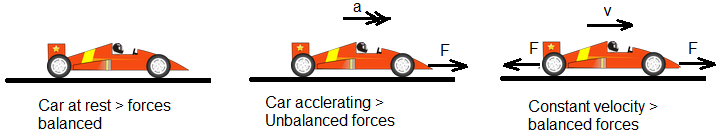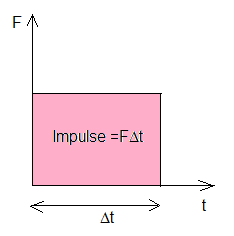# ForcesTake a moment to consider your body right now - the movement of your eyes across the screen, your lungs as they expand and contract, the muscles keeping you upright... and that's before you consider playing sport, or an instrument, or the workings of the brain. Every new movement, direction, squash or stretch requires a force.

Real-world objects are complex, with moving parts, lumps, axles and volume. To simplify things, in Physics most things can be considered as a small ball with forces acting at the centre or contact surfaces.

Key Concepts

Definitions

A force is a push or a pull.

The unit of force is the newton, 1 N, is approximately the force required to hold a mass of 100 g still.

A force is an example of a vector quantity, and so is represented with an arrow. Some forces act when two bodies come into contact; some act at a distance.

Forces are said to be balanced if the resultant force is zero. The body is then in equilibrium.

Equal forces are balanced only if they act in opposite directions.

The resultant can be found of two or more forces in 2-dimensions by vector addition. NB: See Pythagoras and trigonometry for help.

Examples

When a string is attached to body and pulled the force is called tension (T).

Gravitational force (or weight, W) is the force that attracts bodies with mass to the Earth. The force is proportional to the mass.

$$W=mg$$

In this equation, we can consider g to have two possible names:

• Gravitational field strength = 9.81 N kg-1
• Acceleration due to gravity = 9.81 m s-2

Weight acts on the centre of mass of a body.

The normal force (N or R) acts perpendicular to two surfaces that are in contact.

Friction is a force that opposes the motion of a body in contact with a surface.

Friction acts parallel to and along the contact surface. However, it is proportional to the normal force between the surfaces:

$$F=\mu N$$

The constant of proportionality, $$\mu$$ = the coefficient of friction, and varies depending on whether the body is stationary (static friction, $$\mu_s$$) or moving (dynamic friction, $$\mu_d$$).

Buoyancy or upthrust (FB) is the force experienced when a body is totally or partially immersed in a fluid.This is caused by the displacement of fluid particles and is equal to the weight of fluid that would fill the same volume.

FB = weight of fluid displaced

Air resistance is the force that opposes the motion of bodies through the air. This force can be referred to as 'drag' for a body moving through an unspecified fluid.There are different models of air resistance:

• for slow-moving spheres, the force is proportional to velocity, where η is a constant called viscosity and r is the radius

$$F=6\pi \eta vr$$

• for faster-moving objects, the force is proportional to v2

$$F={1\over 2}A\rho C v^2$$

Essentials

Newton's laws of motion

Newton's laws explain the effects of forces on the motion of bodies.

1. The first law states that a body will remain at rest or moving with constant velocity unless acted upon by an unbalanced force.

In simple terms, things don't move or stop moving unless pushed.1. Let's start with a simple statement that applies to bodies with constant mass: The acceleration of a body is proportional to the unbalanced force applied and inversely proportional to its mass.

Considering Newton's first and second laws together, we gather that a body won't move unless pushed, and that for a moving body, the harder you push the faster it will go. This tells us that force and acceleration are related:

$$a\propto{F\over M}$$1 newton is defined as the force required to accelerate a 1 kg mass at a rate of 1 ms-2 and so the constant of proportionality = 1 (unusual in physics!). We can therefore write:

$$F=ma$$

1. If body A exerts a force on body B, then body B will exert an equal and opposite force on body A.

It is important to note that Newton's third law only applies in the following circumstances:

• The forces act on different bodies.
• The forces are the same type.
• The forces are the same size.
• The forces act in opposite directions.

Momentum

Momentum is defined as the product of mass and velocity, and (since m was already taken) is given the symbol p:

$$p=mv$$

The derived SI unit of momentum is kg m s-1 or N s.Can you confirm that kg ms-1 and Ns are dimensionally equivalent?Momentum is a vector quantity, as it is proportional to one other vector quantity.

Impulse is defined as the change of momentum, $$\Delta p =\Delta mv$$

Since forces cause objects to accelerate, and therefore to gain momentum, we can relate force to change in momentum:

$$F = ma = m{(v-u) \over t}$$

$$\Rightarrow F={mv-mu\over t}={\Delta p\over t}$$

The rate of change of momentum of a body is directly proportional to the unbalanced force acting on that body and takes place in the same direction.If we plot a simple force-time graph, we can see that the area under the graph = FΔt. Therefore, the area under a force-time graph is equal to the change in momentum or impulse.

#### Conservation of momentum

For an isolated system of bodies (no external forces) the total momentum is always the same.

$$m_1u_1+m_2u_2=m_1v_1+m_2u_2$$

This applies in each of the following situations:

• Explosion (no initial momentum)
• Elastic collision (no change in total kinetic energy)
• Coalescing collision (reduction in kinetic energy as one object sticks to the other)

Summary

Check out this recap of the concepts so far.

Test Yourself

Use flashcards to practise your recall.

Just for Fun

Check out this πg physics summary.